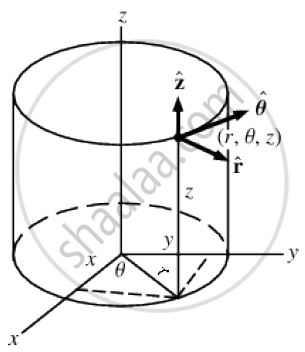# Explain cylindrical co-ordinate system. State the transformation relation between cartesian and cylindrical co-ordinates. - Applied Physics 2

Short Note

Explain cylindrical co-ordinate system.
State the transformation relation between cartesian and cylindrical co-ordinates.

#### Solution1.A cylindrical coordinate system is a three-dimensional coordinate system that specifies point positions by the distance from a chosen reference axis, the direction from the axis relative to a chosen reference direction, and the distance from a chosen reference plane perpendicular to the axis.

2.The latter distance is given as a positive or negative number depending on which side of the reference plane faces the point.

3.The different co-ordinates of cylindrical co-ordinates is shown in the figure above.The angle θ is the angle made by r with X axis.

4.The origin of the system is the point where all three coordinates can be given as zero.

5.This is the intersection between the reference plane and the axis.

6.The distance from the axis may be called the radial distance or radius, while the angular coordinate is sometimes referred to as the angular position or as the azimuth.The radius and the azimuth are together called the polar coordinates, as they correspond to a two-dimensional polar coordinate system in the plane through the point, parallel to the reference plane.

7.The third co-ordinate is called the height or altitude if the reference plane is considered horizontal.

8.Cylindrical coordinates are useful in connection with objects and phenomena that have some rotational symmetry about the longitudinal axis, such as water flow in a straight pipe with round cross-section.

The transformation relation between cartesian and cylindrical co-ordinates are :

(A)Cartesian to cylindrical :

r = sqrt( x^2+y^2)

Ф = tan^(-1)(y/x)
z = z
(B)Cylindrical to cartesian :

x = rcosθ

y = rsinθ
z = z

Concept: Cylindrical and Spherical Coordinate System
Is there an error in this question or solution?